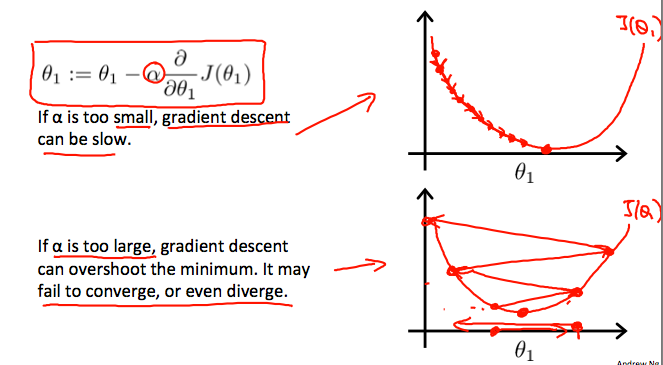• Finding out the best fit line. so it can predict future value.

line equation is :  y = mx +c

M- slope

C- constant

This is our hypothesis :

𝛉0 – constant

𝛉1 – Slope

h(x) – predicted value

Here , we have to tune the value of 𝛉0 and 𝛉1 in such way that our line fit best for the model.

For that we have to customize cost function , by measuring the accuracy of hypothesis.

The cost function J(𝛉)  for Linear regression:

Or

Where, m – no of samples

(½ ) term – convenience for computation of gradient descent

• Ideally line should pass through all the points of dataset , in such case, cost function J(𝛉0,𝛉1) ⇒ 0
• Main objective is to minimise cost function  minimise 𝛉0 , 𝛉1

Linear regression tries to minimize the cost function by finding the proper value of 𝛉0 and 𝛉1 ⇒ by using Gradient Descent Method

For now , Assume  𝛉0 =0

So hypothesis is,  h𝛉(x) = 𝛉1x

And cost function

Here we can see the cost function is dependent on value of 𝛉1

How to reach the minimum of the cost function , when 𝛉1 will equal to 𝛉min.

Suppose , 𝛉1 gets initialized as shown in fig. And cost corresponding to the 𝛉1 is shown in fig as J(𝛉1).

Now , lets update 𝛉1 using Gradient descent.

Here, we apply derivatives on cost function, hence it gives the slope of the curve at that point. This slope is positive , we subtract positive value from actual value of 𝛉1 .

This will force 𝛉1 to move in the left side and slowly diverge to the 𝛉min (cost function is minimum)

• 𝞪 – Learning rate → it decides how much we want to converge in one iteration.

As we move towards the minimum point , slope of the curve getting steeper, that means we are reaching the minimum value , we will have to take small small steps.

Whenever the slope will become zero at min curve then 𝛉1 will not updated.

• The following graph shows that when the slope is negative, the value of Θ1 increases and when it is positive, the value of θ1 decreases.
• we should adjust our parameter α to ensure that the gradient descent algorithm converges in a reasonable time. Failure to converge or too much time to obtain the minimum value imply that our step size is wrong.
•Derivative of Cost Function :

Let’s differentiate the cost function:

if we start with a guess for our hypothesis and then repeatedly apply these gradient descent equations, our hypothesis will become more and more accurate.